# Zenith Bank Lowered Loan Exposure paid 30k Interim Cash Dividend-H1-2018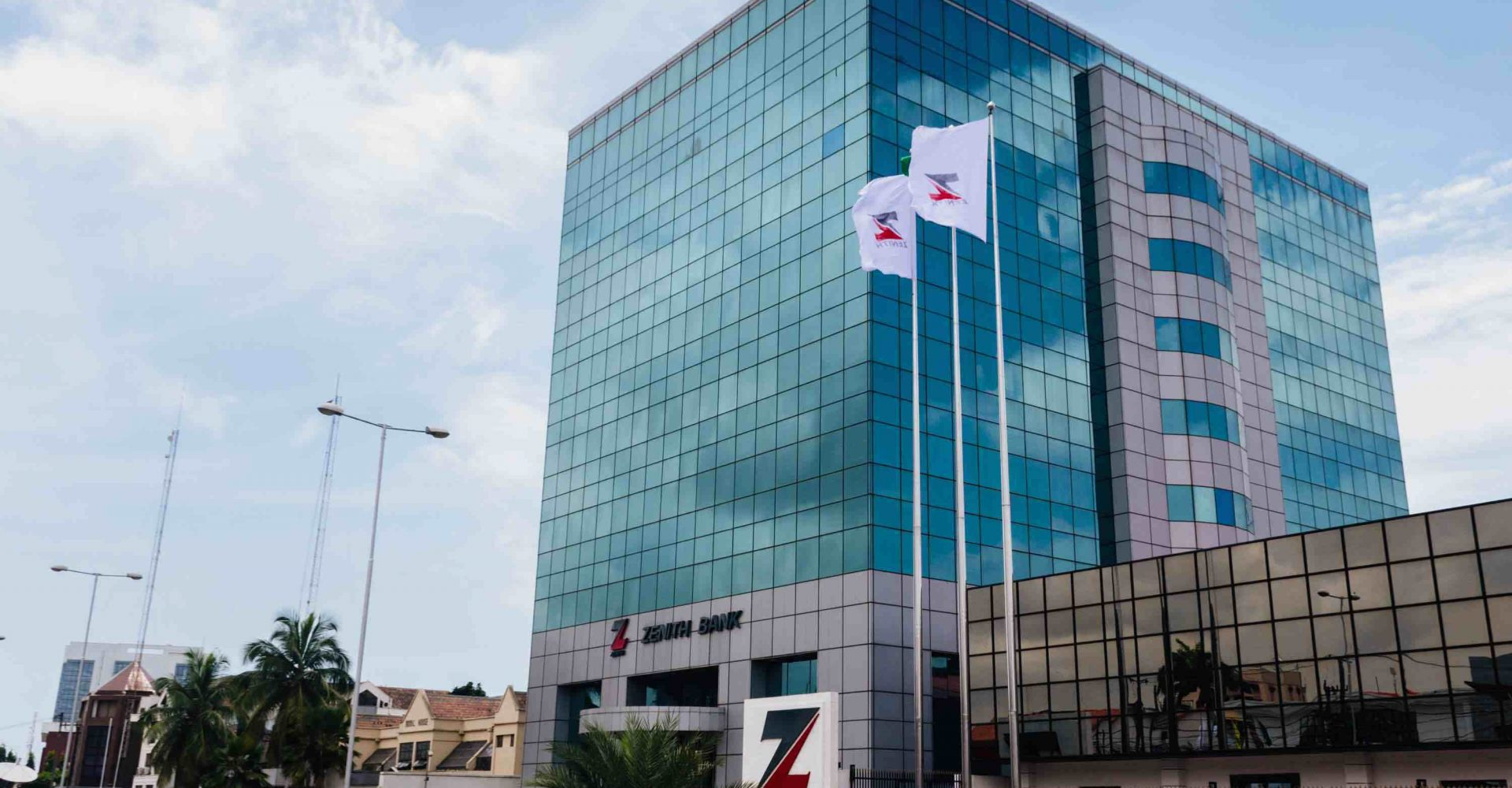Coy: Zenith Bank Plc

Current Market Price:

Year High: N34.20

Year Low: N 19.60

Fair Value: N40.89

Key Financial Ratios

• Interim Dividend for the period is 30k as against the 25k paid in 2017 Half year financial performance
• Thus, the Payout Ratio is same as 11.52% (H1-2017-10.42%)
• Sustainable Growth rate is currently estimated at 10.05%
• Non Performing loan ratio as at the end of the half year financial performance of 2018 is 5.51% as against the 3.28% posted in its full year financial performance for 2017.
• The five (5) years average dividend yield of Zenith Bank Plc has been estimated at 10% above the industrial average of 2.21%
• Similarly, the five (5) years dividend growth rate is currently estimated at 11.03% lower than the estimated industrial average of 25.77%
• Five (5) years average effective tax rate is currently estimated at 15.11%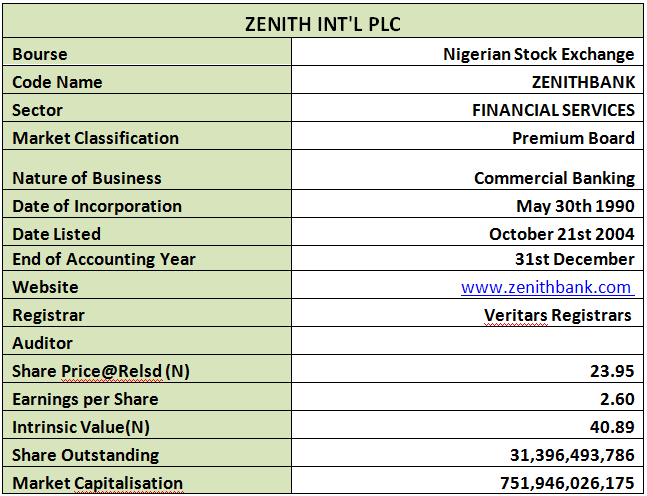Company Figures

• Reported Gross Earnings for the half year 2018 financial activities is 15.31% below the corresponding quarter in 2017’s figure. Please note that N322.201 billion was reported as Gross Earnings as against the previous N380.440 billion
• 19% same as N74.709 billion of the Gross Earnings was reported as Interest Expense for the period as against N123.29 billion same as 32.41% that was reported last H1 financials
• Nevertheless, the Profit before Tax (PBT) reported for the period was N107.35 billion, this is 16.46% above the N92.18 billion achieved in the corresponding period of 2018
• Profit after Tax (PAT) equally stemmed above that of last year by 8.52%, having moved from N75.317 billion to N81.737 billion
• Total Assets reported for the period stood at N5,256.46 trillion compared to the N4,927.36 trillion in 2017
• Total Liabilities stood at 7.82% above same quarter in 2017
• Total deposits from customers through the reported six months was estimated at N3,165.95 trillion this a marginal growth of 6.42% above the 2017 deposit of N2,972.93 trillion
• Perhaps due to conservative approach, the amount given out as Loans and Advances reduced by 14.36% when compared with the reported value in 2017
• Due to marginal difference between the Total Assets and Total Liabilities for the two periods compared in this report, Net Assets estimated for the reported period only inched above that of the similar quarter of 2017 by 2%.
• See below table for details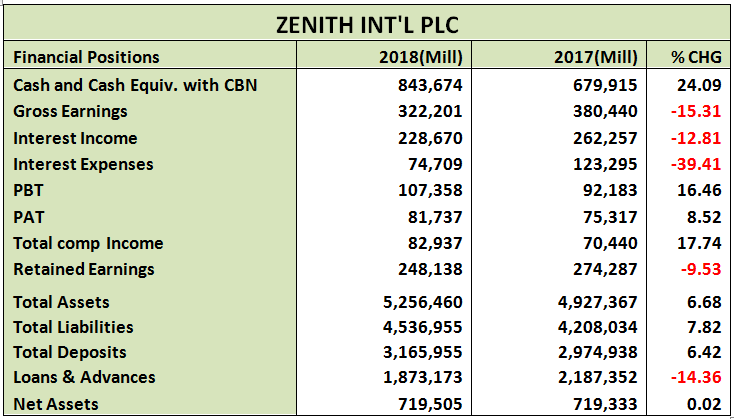Volatility Ratios

• Although the estimated beta value for Zenith Bank shares stood fairly below the industrial average, it is well above the market beta. This signifies its liquidity and patronage by the investing public
• Although almost irrelevant since we analyze a financial institution whose major business is to collect deposit (mostly reported under liability) we estimated Debt to Equity ratio as 106.74% well above the industrial average of 25.30%Profitability Ratios

• Interest Expense to Gross Earnings is presently estimated at 23.19% this is 28.45% lower than the 32.41% estimated in 2017 half year result
• PBT margin stood at 33.32% as against 24.23% last year, this is 37.51% improvement
• Similarly, Profit margin looks up against last year. We have estimated 25.37% margin from Gross Earnings as against the previous estimate of 19.89%
• Return on Average Equity is now 11.36% compared to the 10.47% returns achieved in the first six months of 2017
• Return on Average Assets only differs marginally by 1.73%, moving from 1.53% to 1.55%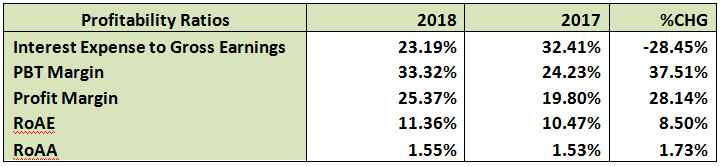Efficiency Ratio

• Gross Earnings to Total Assets is estimated at 6.13% this is 20.61% lower than the estimate in 2017
• Similarly, Gross Earnings to Equity is now 44.78% as against the 52.89% estimated from 2017 half year financial statistics
• Financial Leverage is 7.31x as against 6.85x, this is an estimate of the number of times the total assets replicates the equity, in other words the ratio got better
• It was also established that 59.17% of the Total Deposit was given out as Loan and Advances during the period under estimate this is 19.53% lower than the 75.53% given out during the first six months of 2017
• Meanwhile, Loan and Advances is same as 35.64% of the Total Assets this is 19.72% lower than the 44.39% of last half year. This shows a controlled/reduced risk compared to 2017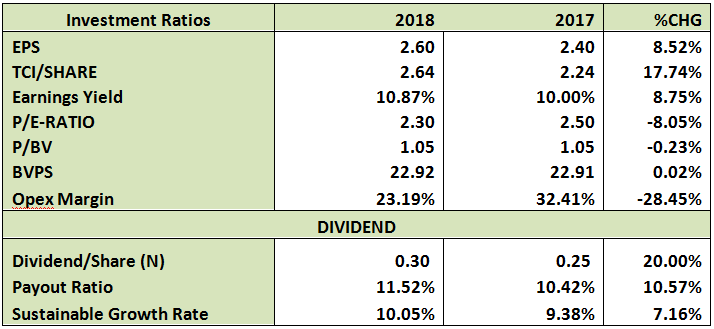Valuation

Our attempt to place a fair value on Zenith Bank took us through several valuation methods after which we settled for the constant perpetual dividend growth rate. We do not expect galloping growth in dividend since this has experienced appreciable growth in few years prior, so we make use of 2.758% as our growth rate. To arrive at this value, we estimated five years dividend growth rate at 11.03%, and we make use of the average. It was also assumed that the final dividend will be around N2.50, and discounted at 10%. On this strength, we have placed a fair value of N40.89.

This site uses Akismet to reduce spam. Learn how your comment data is processed.

# Zenith Bank Lowered Loan Exposure paid 30k Interim Cash Dividend-H1-2018Coy: Zenith Bank Plc

Current Market Price:

Year High: N34.20

Year Low: N 19.60

Fair Value: N40.89

Key Financial Ratios

• Interim Dividend for the period is 30k as against the 25k paid in 2017 Half year financial performance
• Thus, the Payout Ratio is same as 11.52% (H1-2017-10.42%)
• Sustainable Growth rate is currently estimated at 10.05%
• Non Performing loan ratio as at the end of the half year financial performance of 2018 is 5.51% as against the 3.28% posted in its full year financial performance for 2017.
• The five (5) years average dividend yield of Zenith Bank Plc has been estimated at 10% above the industrial average of 2.21%
• Similarly, the five (5) years dividend growth rate is currently estimated at 11.03% lower than the estimated industrial average of 25.77%
• Five (5) years average effective tax rate is currently estimated at 15.11%Company Figures

• Reported Gross Earnings for the half year 2018 financial activities is 15.31% below the corresponding quarter in 2017’s figure. Please note that N322.201 billion was reported as Gross Earnings as against the previous N380.440 billion
• 19% same as N74.709 billion of the Gross Earnings was reported as Interest Expense for the period as against N123.29 billion same as 32.41% that was reported last H1 financials
• Nevertheless, the Profit before Tax (PBT) reported for the period was N107.35 billion, this is 16.46% above the N92.18 billion achieved in the corresponding period of 2018
• Profit after Tax (PAT) equally stemmed above that of last year by 8.52%, having moved from N75.317 billion to N81.737 billion
• Total Assets reported for the period stood at N5,256.46 trillion compared to the N4,927.36 trillion in 2017
• Total Liabilities stood at 7.82% above same quarter in 2017
• Total deposits from customers through the reported six months was estimated at N3,165.95 trillion this a marginal growth of 6.42% above the 2017 deposit of N2,972.93 trillion
• Perhaps due to conservative approach, the amount given out as Loans and Advances reduced by 14.36% when compared with the reported value in 2017
• Due to marginal difference between the Total Assets and Total Liabilities for the two periods compared in this report, Net Assets estimated for the reported period only inched above that of the similar quarter of 2017 by 2%.
• See below table for detailsVolatility Ratios

• Although the estimated beta value for Zenith Bank shares stood fairly below the industrial average, it is well above the market beta. This signifies its liquidity and patronage by the investing public
• Although almost irrelevant since we analyze a financial institution whose major business is to collect deposit (mostly reported under liability) we estimated Debt to Equity ratio as 106.74% well above the industrial average of 25.30%Profitability Ratios

• Interest Expense to Gross Earnings is presently estimated at 23.19% this is 28.45% lower than the 32.41% estimated in 2017 half year result
• PBT margin stood at 33.32% as against 24.23% last year, this is 37.51% improvement
• Similarly, Profit margin looks up against last year. We have estimated 25.37% margin from Gross Earnings as against the previous estimate of 19.89%
• Return on Average Equity is now 11.36% compared to the 10.47% returns achieved in the first six months of 2017
• Return on Average Assets only differs marginally by 1.73%, moving from 1.53% to 1.55%Efficiency Ratio

• Gross Earnings to Total Assets is estimated at 6.13% this is 20.61% lower than the estimate in 2017
• Similarly, Gross Earnings to Equity is now 44.78% as against the 52.89% estimated from 2017 half year financial statistics
• Financial Leverage is 7.31x as against 6.85x, this is an estimate of the number of times the total assets replicates the equity, in other words the ratio got better
• It was also established that 59.17% of the Total Deposit was given out as Loan and Advances during the period under estimate this is 19.53% lower than the 75.53% given out during the first six months of 2017
• Meanwhile, Loan and Advances is same as 35.64% of the Total Assets this is 19.72% lower than the 44.39% of last half year. This shows a controlled/reduced risk compared to 2017Valuation

Our attempt to place a fair value on Zenith Bank took us through several valuation methods after which we settled for the constant perpetual dividend growth rate. We do not expect galloping growth in dividend since this has experienced appreciable growth in few years prior, so we make use of 2.758% as our growth rate. To arrive at this value, we estimated five years dividend growth rate at 11.03%, and we make use of the average. It was also assumed that the final dividend will be around N2.50, and discounted at 10%. On this strength, we have placed a fair value of N40.89.

This site uses Akismet to reduce spam. Learn how your comment data is processed.